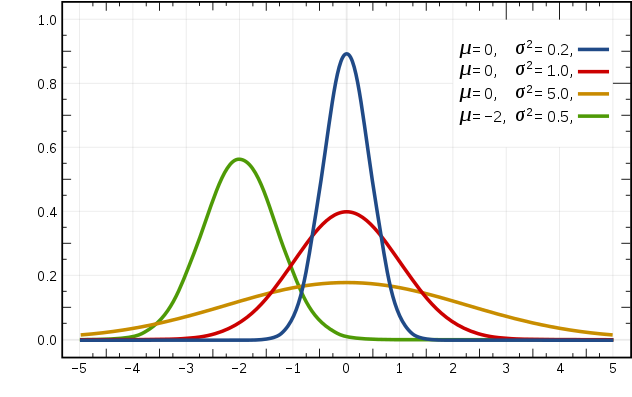# 机器学习| 朴素贝叶斯详解 (Python 语言描述)

## 朴素贝叶斯基础

### 基本概念

#### 条件概率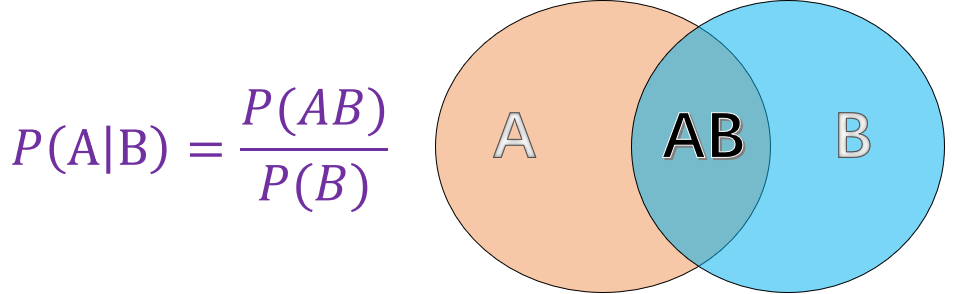• $$P(A)$$ 表示 $$A$$ 事件发生的概率。
• $$P(B)$$ 表示 $$B$$ 事件发生的概率。
• $$P(AB)$$ 表示 $$A, B$$ 事件同时发生的概率。

#### 贝叶斯定理

$$P(B \mid A)=\frac{P(AB)}{P(A)} \tag1$$

$$P(AB)=P(A \mid B)*P(B) \tag2$$

$$P(B \mid A)=\frac{P(AB)}{P(A)}=\frac{P(A \mid B)*P(B)}{P(A)} \tag{3}$$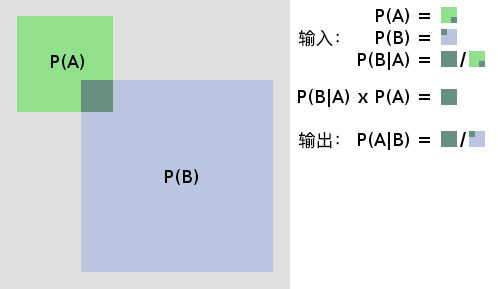### 什么是朴素贝叶斯

$$P(B \mid A)=\frac{P(A \mid B)*P(B)}{P(A)} \tag{4}$$

$$P(类别 \mid 特征)=\frac{P(特征 \mid 类别) * P(类别)}{P(特征)} \tag{5}$$

## 朴素贝叶斯算法实现

### 生成示例数据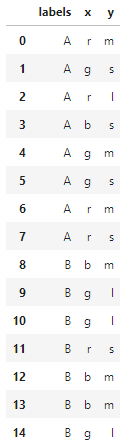### 参数估计

#### 极大似然估计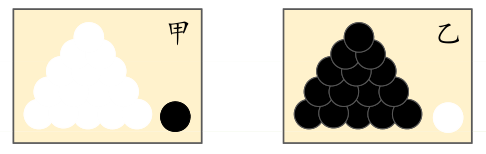$$P(y_{i}=c_{k})=\frac{\sum_{N}^{i=1}I(y_{i}=c_{k})}{N},k=1,2,3,…,m \tag{6}$$

$$P(特征 \mid 种类)$$ 由于公式较为繁琐这里先不给出，直接用叙述的方式能更清晰地帮助理解：

#### 贝叶斯估计

$$P(y_{i}=c_{k})=\frac{\sum_{N}^{i=1}I(y_{i}=c_{k})+\lambda }{N+k\lambda} \tag{8}$$

### 朴素贝叶斯的三种常见模型

#### 伯努利模型

• 当特征值 $$x_{i}=1$$ 时，$$P(x_{i} \mid y_{k})=P(x_{i}=1 \mid y_{k})$$;
• 当特征值 $$x_{i}=0$$ 时，$$P(x_{i} \mid y_{k})=P(x_{i}=0 \mid y_{k})$$。

#### 高斯模型

$$P(x_{i}|y_{k})=\frac{1}{\sqrt{2\pi}\sigma_{y_{k},i}}exp(-\frac{(x-\mu_{y_{k},i}) ^{2}}{2\sigma ^{2}_{y_{k}},i})$$

• $$\mu_{y_{k},i}$$ 表示类别为 $$y_{k}$$ 的样本中，第 i 维特征的均值。
• $$\sigma ^{2}_{y_{k}},i$$ 表示类别为 $$y_{k}$$ 的样本中，第 i 维特征的方差。